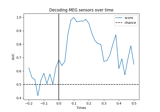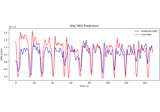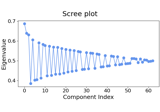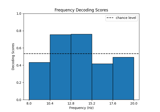# mne.decoding.CSP#

class mne.decoding.CSP(n_components=4, reg=None, log=None, cov_est='concat', transform_into='average_power', norm_trace=False, cov_method_params=None, rank=None, component_order='mutual_info')[source]#

M/EEG signal decomposition using the Common Spatial Patterns (CSP).

This class can be used as a supervised decomposition to estimate spatial filters for feature extraction. CSP in the context of EEG was first described in 1; a comprehensive tutorial on CSP can be found in 2. Multi-class solving is implemented from 3.

Parameters
n_components`int` (default 4)

The number of components to decompose M/EEG signals. This number should be set by cross-validation.

reg

If not None (same as `'empirical'`, default), allow regularization for covariance estimation. If float (between 0 and 1), shrinkage is used. For str values, `reg` will be passed as `method` to `mne.compute_covariance()`.

log

If `transform_into` equals `'average_power'` and `log` is None or True, then apply a log transform to standardize features, else features are z-scored. If `transform_into` is `'csp_space'`, `log` must be None.

cov_est‘concat’ | ‘epoch’ (default ‘concat’)

If `'concat'`, covariance matrices are estimated on concatenated epochs for each class. If `'epoch'`, covariance matrices are estimated on each epoch separately and then averaged over each class.

transform_into‘average_power’ | ‘csp_space’ (default ‘average_power’)

If ‘average_power’ then `self.transform` will return the average power of each spatial filter. If `'csp_space'`, `self.transform` will return the data in CSP space.

norm_trace`bool` (default `False`)

Normalize class covariance by its trace. Trace normalization is a step of the original CSP algorithm 1 to eliminate magnitude variations in the EEG between individuals. It is not applied in more recent work 2, 3 and can have a negative impact on pattern order.

cov_method_params

Parameters to pass to `mne.compute_covariance()`.

New in v0.16.

rank`None` | ‘info’ | ‘full’ | `dict`

This controls the rank computation that can be read from the measurement info or estimated from the data. When a noise covariance is used for whitening, this should reflect the rank of that covariance, otherwise amplification of noise components can occur in whitening (e.g., often during source localization).

`None`

The rank will be estimated from the data after proper scaling of different channel types.

`'info'`

The rank is inferred from `info`. If data have been processed with Maxwell filtering, the Maxwell filtering header is used. Otherwise, the channel counts themselves are used. In both cases, the number of projectors is subtracted from the (effective) number of channels in the data. For example, if Maxwell filtering reduces the rank to 68, with two projectors the returned value will be 66.

`'full'`

The rank is assumed to be full, i.e. equal to the number of good channels. If a `Covariance` is passed, this can make sense if it has been (possibly improperly) regularized without taking into account the true data rank.

`dict`

Calculate the rank only for a subset of channel types, and explicitly specify the rank for the remaining channel types. This can be extremely useful if you already know the rank of (part of) your data, for instance in case you have calculated it earlier.

This parameter must be a dictionary whose keys correspond to channel types in the data (e.g. `'meg'`, `'mag'`, `'grad'`, `'eeg'`), and whose values are integers representing the respective ranks. For example, `{'mag': 90, 'eeg': 45}` will assume a rank of `90` and `45` for magnetometer data and EEG data, respectively.

The ranks for all channel types present in the data, but not specified in the dictionary will be estimated empirically. That is, if you passed a dataset containing magnetometer, gradiometer, and EEG data together with the dictionary from the previous example, only the gradiometer rank would be determined, while the specified magnetometer and EEG ranks would be taken for granted.

The default is `None`.

New in v0.17.

component_order‘mutual_info’ | ‘alternate’ (default ‘mutual_info’)

If `'mutual_info'` order components by decreasing mutual information (in the two-class case this uses a simplification which orders components by decreasing absolute deviation of the eigenvalues from 0.5 4). For the two-class case, `'alternate'` orders components by starting with the largest eigenvalue, followed by the smallest, the second-to-largest, the second-to-smallest, and so on 2.

New in v0.21.

References

1(1,2)

Zoltan J. Koles, Michael S. Lazar, and Steven Z. Zhou. Spatial patterns underlying population differences in the background EEG. Brain Topography, 2(4):275–284, 1990. doi:10.1007/BF01129656.

2(1,2,3)

Benjamin Blankertz, Ryota Tomioka, Steven Lemm, Motoaki Kawanabe, and Klaus-Robert Müller. Optimizing spatial filters for robust EEG single-trial analysis. IEEE Signal Processing Magazine, 25(1):41–56, 2008. doi:10.1109/MSP.2008.4408441.

3(1,2)

Moritz Grosse-Wentrup and Martin Buss. Multiclass common spatial patterns and information theoretic feature extraction. IEEE Transactions on Biomedical Engineering, 55(8):1991–2000, 2008. doi:10.1109/TBME.2008.921154.

4

Alexandre Barachant, Stephane Bonnet, Marco Congedo, and Christian Jutten. Common spatial pattern revisited by Riemannian geometry. In 2010 IEEE International Workshop on Multimedia Signal Processing, 472–476. 2010. doi:10.1109/MMSP.2010.5662067.

Attributes
filters_`ndarray`, shape (n_channels, n_channels)

If fit, the CSP components used to decompose the data, else None.

patterns_`ndarray`, shape (n_channels, n_channels)

If fit, the CSP patterns used to restore M/EEG signals, else None.

mean_`ndarray`, shape (n_components,)

If fit, the mean squared power for each component.

std_`ndarray`, shape (n_components,)

If fit, the std squared power for each component.

Methods

 `fit`(X, y) Estimate the CSP decomposition on epochs. `fit_transform`(X, y, **fit_params) Fit to data, then transform it. `get_params`([deep]) Get parameters for this estimator. `plot_filters`(info[, components, average, ...]) Plot topographic filters of components. `plot_patterns`(info[, components, average, ...]) Plot topographic patterns of components. `set_params`(**params) Set the parameters of this estimator. Estimate epochs sources given the CSP filters.
fit(X, y)[source]#

Estimate the CSP decomposition on epochs.

Parameters
X`ndarray`, shape (n_epochs, n_channels, n_times)

The data on which to estimate the CSP.

y`array`, shape (n_epochs,)

The class for each epoch.

Returns
selfinstance of `CSP`

Returns the modified instance.

Examples using `fit`:Decoding (MVPA)

Decoding (MVPA)Continuous Target Decoding with SPoC

Continuous Target Decoding with SPoC
fit_transform(X, y, **fit_params)[source]#

Fit to data, then transform it.

Fits transformer to `X` and `y` with optional parameters `fit_params`, and returns a transformed version of `X`.

Parameters
X`array`, shape (n_samples, n_features)

Training set.

y`array`, shape (n_samples,)

Target values or class labels.

**fit_params`dict`

Additional fitting parameters passed to the `fit` method..

Returns
X_new`array`, shape (n_samples, n_features_new)

Transformed array.

Examples using `fit_transform`:Motor imagery decoding from EEG data using the Common Spatial Pattern (CSP)

Motor imagery decoding from EEG data using the Common Spatial Pattern (CSP)
get_params(deep=True)[source]#

Get parameters for this estimator.

Parameters
deep`bool`, optional

If True, will return the parameters for this estimator and contained subobjects that are estimators.

Returns
params`dict`

Parameter names mapped to their values.

plot_filters(info, components=None, *, average=None, ch_type=None, scalings=None, sensors=True, show_names=False, mask=None, mask_params=None, contours=6, outlines='head', sphere=None, image_interp='cubic', extrapolate='auto', border='mean', res=64, size=1, cmap='RdBu_r', vlim=(None, None), cnorm=None, colorbar=True, cbar_fmt='%3.1f', units=None, axes=None, name_format='CSP%01d', nrows=1, ncols='auto', show=True)[source]#

Plot topographic filters of components.

The filters are used to extract discriminant neural sources from the measured data (a.k.a. the backward model).

Parameters
info`mne.Info`

The `mne.Info` object with information about the sensors and methods of measurement. Used for fitting. If not available, consider using `mne.create_info()`.

components

The patterns to plot. If `None`, all components will be shown.

average`float` | array_like of `float`, shape (n_times,) | `None`

The time window (in seconds) around a given time point to be used for averaging. For example, 0.2 would translate into a time window that starts 0.1 s before and ends 0.1 s after the given time point. If the time window exceeds the duration of the data, it will be clipped. Different time windows (one per time point) can be provided by passing an `array-like` object (e.g., `[0.1, 0.2, 0.3]`). If `None` (default), no averaging will take place.

Changed in version 1.1: Support for `array-like` input.

ch_type‘mag’ | ‘grad’ | ‘planar1’ | ‘planar2’ | ‘eeg’ | `None`

The channel type to plot. For `'grad'`, the gradiometers are collected in pairs and the RMS for each pair is plotted. If `None` the first available channel type from order shown above is used. Defaults to `None`.

scalings

The scalings of the channel types to be applied for plotting. If None, defaults to `dict(eeg=1e6, grad=1e13, mag=1e15)`.

sensors

Whether to add markers for sensor locations. If `str`, should be a valid matplotlib format string (e.g., `'r+'` for red plusses, see the Notes section of `plot()`). If `True` (the default), black circles will be used.

show_names

If `True`, show channel names next to each sensor marker. If callable, channel names will be formatted using the callable; e.g., to delete the prefix ‘MEG ‘ from all channel names, pass the function `lambda x: x.replace('MEG ', '')`. If `mask` is not `None`, only non-masked sensor names will be shown.

mask`ndarray` of `bool`, shape (n_channels, n_patterns) | `None`

Array indicating channel-pattern combinations to highlight with a distinct plotting style. Array elements set to `True` will be plotted with the parameters given in `mask_params`. Defaults to `None`, equivalent to an array of all `False` elements.

Additional plotting parameters for plotting significant sensors. Default (None) equals:

```dict(marker='o', markerfacecolor='w', markeredgecolor='k',
linewidth=0, markersize=4)
```
contours

The number of contour lines to draw. If `0`, no contours will be drawn. If a positive integer, that number of contour levels are chosen using the matplotlib tick locator (may sometimes be inaccurate, use array for accuracy). If array-like, the array values are used as the contour levels. The values should be in µV for EEG, fT for magnetometers and fT/m for gradiometers. If `colorbar=True`, the colorbar will have ticks corresponding to the contour levels. Default is `6`.

outlines‘head’ | `dict` | `None`

The outlines to be drawn. If ‘head’, the default head scheme will be drawn. If dict, each key refers to a tuple of x and y positions, the values in ‘mask_pos’ will serve as image mask. Alternatively, a matplotlib patch object can be passed for advanced masking options, either directly or as a function that returns patches (required for multi-axis plots). If None, nothing will be drawn. Defaults to ‘head’.

sphere`float` | array_like | instance of `ConductorModel` | `None` | ‘auto’ | ‘eeglab’

The sphere parameters to use for the head outline. Can be array-like of shape (4,) to give the X/Y/Z origin and radius in meters, or a single float to give just the radius (origin assumed 0, 0, 0). Can also be an instance of a spherical `ConductorModel` to use the origin and radius from that object. If `'auto'` the sphere is fit to digitization points. If `'eeglab'` the head circle is defined by EEG electrodes `'Fpz'`, `'Oz'`, `'T7'`, and `'T8'` (if `'Fpz'` is not present, it will be approximated from the coordinates of `'Oz'`). `None` (the default) is equivalent to `'auto'` when enough extra digitization points are available, and (0, 0, 0, 0.095) otherwise.

New in v0.20.

Changed in version 1.1: Added `'eeglab'` option.

image_interp`str`

The image interpolation to be used. Options are `'cubic'` (default) to use `scipy.interpolate.CloughTocher2DInterpolator`, `'nearest'` to use `scipy.spatial.Voronoi` or `'linear'` to use `scipy.interpolate.LinearNDInterpolator`.

extrapolate`str`

Options:

• `'box'`

Extrapolate to four points placed to form a square encompassing all data points, where each side of the square is three times the range of the data in the respective dimension.

• `'local'` (default for MEG sensors)

Extrapolate only to nearby points (approximately to points closer than median inter-electrode distance). This will also set the mask to be polygonal based on the convex hull of the sensors.

• `'head'` (default for non-MEG sensors)

Extrapolate out to the edges of the clipping circle. This will be on the head circle when the sensors are contained within the head circle, but it can extend beyond the head when sensors are plotted outside the head circle.

New in v1.3.

border`float` | ‘mean’

Value to extrapolate to on the topomap borders. If `'mean'` (default), then each extrapolated point has the average value of its neighbours.

New in v1.3.

res`int`

The resolution of the topomap image (number of pixels along each side).

size`float`

Side length of each subplot in inches.

cmapmatplotlib colormap | (colormap, `bool`) | ‘interactive’ | `None`

Colormap to use. If `tuple`, the first value indicates the colormap to use and the second value is a boolean defining interactivity. In interactive mode the colors are adjustable by clicking and dragging the colorbar with left and right mouse button. Left mouse button moves the scale up and down and right mouse button adjusts the range. Hitting space bar resets the range. Up and down arrows can be used to change the colormap. If `None`, `'Reds'` is used for data that is either all-positive or all-negative, and `'RdBu_r'` is used otherwise. `'interactive'` is equivalent to `(None, True)`. Defaults to `None`.

Warning

Interactive mode works smoothly only for a small amount of topomaps. Interactive mode is disabled by default for more than 2 topomaps.

vlim`tuple` of length 2 | ‘joint’

Colormap limits to use. If a `tuple` of floats, specifies the lower and upper bounds of the colormap (in that order); providing `None` for either entry will set the corresponding boundary at the min/max of the data (separately for each topomap). Elements of the `tuple` may also be callable functions which take in a `NumPy array` and return a scalar. If `vlim='joint'`, will compute the colormap limits jointly across all topomaps of the same channel type, using the min/max of the data for that channel type. Defaults to `(None, None)`.

New in v1.3.

cnorm

How to normalize the colormap. If `None`, standard linear normalization is performed. If not `None`, `vmin` and `vmax` will be ignored. See Matplotlib docs for more details on colormap normalization, and the ERDs example for an example of its use.

New in v1.3.

colorbar`bool`

Plot a colorbar in the rightmost column of the figure.

cbar_fmt`str`

Formatting string for colorbar tick labels. See Format Specification Mini-Language for details.

units

The units to use for the colorbar label. Ignored if `colorbar=False`. If `None` the label will be “AU” indicating arbitrary units. Default is `None`.

axesinstance of `Axes` | `list` of `Axes` | `None`

The axes to plot to. If `None`, a new `Figure` will be created with the correct number of axes. If `Axes` are provided (either as a single instance or a `list` of axes), the number of axes provided must match the number of `times` provided (unless `times` is `None`).Default is `None`.

name_format`str`

String format for topomap values. Defaults to “CSP%01d”.

nrows, ncols`int` | ‘auto’

The number of rows and columns of topographies to plot. If either `nrows` or `ncols` is `'auto'`, the necessary number will be inferred. Defaults to `nrows=1, ncols='auto'`.

New in v1.3.

show`bool`

Show the figure if `True`.

Returns
figinstance of `matplotlib.figure.Figure`

The figure.

Examples using `plot_filters`:Decoding (MVPA)

Decoding (MVPA)
plot_patterns(info, components=None, *, average=None, ch_type=None, scalings=None, sensors=True, show_names=False, mask=None, mask_params=None, contours=6, outlines='head', sphere=None, image_interp='cubic', extrapolate='auto', border='mean', res=64, size=1, cmap='RdBu_r', vlim=(None, None), cnorm=None, colorbar=True, cbar_fmt='%3.1f', units=None, axes=None, name_format='CSP%01d', nrows=1, ncols='auto', show=True)[source]#

Plot topographic patterns of components.

The patterns explain how the measured data was generated from the neural sources (a.k.a. the forward model).

Parameters
info`mne.Info`

The `mne.Info` object with information about the sensors and methods of measurement. Used for fitting. If not available, consider using `mne.create_info()`.

components

The patterns to plot. If `None`, all components will be shown.

average`float` | array_like of `float`, shape (n_times,) | `None`

The time window (in seconds) around a given time point to be used for averaging. For example, 0.2 would translate into a time window that starts 0.1 s before and ends 0.1 s after the given time point. If the time window exceeds the duration of the data, it will be clipped. Different time windows (one per time point) can be provided by passing an `array-like` object (e.g., `[0.1, 0.2, 0.3]`). If `None` (default), no averaging will take place.

Changed in version 1.1: Support for `array-like` input.

ch_type‘mag’ | ‘grad’ | ‘planar1’ | ‘planar2’ | ‘eeg’ | `None`

The channel type to plot. For `'grad'`, the gradiometers are collected in pairs and the RMS for each pair is plotted. If `None` the first available channel type from order shown above is used. Defaults to `None`.

scalings

The scalings of the channel types to be applied for plotting. If None, defaults to `dict(eeg=1e6, grad=1e13, mag=1e15)`.

sensors

Whether to add markers for sensor locations. If `str`, should be a valid matplotlib format string (e.g., `'r+'` for red plusses, see the Notes section of `plot()`). If `True` (the default), black circles will be used.

show_names

If `True`, show channel names next to each sensor marker. If callable, channel names will be formatted using the callable; e.g., to delete the prefix ‘MEG ‘ from all channel names, pass the function `lambda x: x.replace('MEG ', '')`. If `mask` is not `None`, only non-masked sensor names will be shown.

mask`ndarray` of `bool`, shape (n_channels, n_patterns) | `None`

Array indicating channel-pattern combinations to highlight with a distinct plotting style. Array elements set to `True` will be plotted with the parameters given in `mask_params`. Defaults to `None`, equivalent to an array of all `False` elements.

Additional plotting parameters for plotting significant sensors. Default (None) equals:

```dict(marker='o', markerfacecolor='w', markeredgecolor='k',
linewidth=0, markersize=4)
```
contours

The number of contour lines to draw. If `0`, no contours will be drawn. If a positive integer, that number of contour levels are chosen using the matplotlib tick locator (may sometimes be inaccurate, use array for accuracy). If array-like, the array values are used as the contour levels. The values should be in µV for EEG, fT for magnetometers and fT/m for gradiometers. If `colorbar=True`, the colorbar will have ticks corresponding to the contour levels. Default is `6`.

outlines‘head’ | `dict` | `None`

The outlines to be drawn. If ‘head’, the default head scheme will be drawn. If dict, each key refers to a tuple of x and y positions, the values in ‘mask_pos’ will serve as image mask. Alternatively, a matplotlib patch object can be passed for advanced masking options, either directly or as a function that returns patches (required for multi-axis plots). If None, nothing will be drawn. Defaults to ‘head’.

sphere`float` | array_like | instance of `ConductorModel` | `None` | ‘auto’ | ‘eeglab’

The sphere parameters to use for the head outline. Can be array-like of shape (4,) to give the X/Y/Z origin and radius in meters, or a single float to give just the radius (origin assumed 0, 0, 0). Can also be an instance of a spherical `ConductorModel` to use the origin and radius from that object. If `'auto'` the sphere is fit to digitization points. If `'eeglab'` the head circle is defined by EEG electrodes `'Fpz'`, `'Oz'`, `'T7'`, and `'T8'` (if `'Fpz'` is not present, it will be approximated from the coordinates of `'Oz'`). `None` (the default) is equivalent to `'auto'` when enough extra digitization points are available, and (0, 0, 0, 0.095) otherwise.

New in v0.20.

Changed in version 1.1: Added `'eeglab'` option.

image_interp`str`

The image interpolation to be used. Options are `'cubic'` (default) to use `scipy.interpolate.CloughTocher2DInterpolator`, `'nearest'` to use `scipy.spatial.Voronoi` or `'linear'` to use `scipy.interpolate.LinearNDInterpolator`.

extrapolate`str`

Options:

• `'box'`

Extrapolate to four points placed to form a square encompassing all data points, where each side of the square is three times the range of the data in the respective dimension.

• `'local'` (default for MEG sensors)

Extrapolate only to nearby points (approximately to points closer than median inter-electrode distance). This will also set the mask to be polygonal based on the convex hull of the sensors.

• `'head'` (default for non-MEG sensors)

Extrapolate out to the edges of the clipping circle. This will be on the head circle when the sensors are contained within the head circle, but it can extend beyond the head when sensors are plotted outside the head circle.

New in v1.3.

border`float` | ‘mean’

Value to extrapolate to on the topomap borders. If `'mean'` (default), then each extrapolated point has the average value of its neighbours.

New in v1.3.

res`int`

The resolution of the topomap image (number of pixels along each side).

size`float`

Side length of each subplot in inches.

cmapmatplotlib colormap | (colormap, `bool`) | ‘interactive’ | `None`

Colormap to use. If `tuple`, the first value indicates the colormap to use and the second value is a boolean defining interactivity. In interactive mode the colors are adjustable by clicking and dragging the colorbar with left and right mouse button. Left mouse button moves the scale up and down and right mouse button adjusts the range. Hitting space bar resets the range. Up and down arrows can be used to change the colormap. If `None`, `'Reds'` is used for data that is either all-positive or all-negative, and `'RdBu_r'` is used otherwise. `'interactive'` is equivalent to `(None, True)`. Defaults to `None`.

Warning

Interactive mode works smoothly only for a small amount of topomaps. Interactive mode is disabled by default for more than 2 topomaps.

vlim`tuple` of length 2

Colormap limits to use. If a `tuple` of floats, specifies the lower and upper bounds of the colormap (in that order); providing `None` for either entry will set the corresponding boundary at the min/max of the data. Defaults to `(None, None)`.

New in v1.3.

cnorm

How to normalize the colormap. If `None`, standard linear normalization is performed. If not `None`, `vmin` and `vmax` will be ignored. See Matplotlib docs for more details on colormap normalization, and the ERDs example for an example of its use.

New in v1.3.

colorbar`bool`

Plot a colorbar in the rightmost column of the figure.

cbar_fmt`str`

Formatting string for colorbar tick labels. See Format Specification Mini-Language for details.

units

The units to use for the colorbar label. Ignored if `colorbar=False`. If `None` the label will be “AU” indicating arbitrary units. Default is `None`.

axesinstance of `Axes` | `list` of `Axes` | `None`

The axes to plot to. If `None`, a new `Figure` will be created with the correct number of axes. If `Axes` are provided (either as a single instance or a `list` of axes), the number of axes provided must match the number of `times` provided (unless `times` is `None`).Default is `None`.

name_format`str`

String format for topomap values. Defaults to “CSP%01d”.

nrows, ncols`int` | ‘auto’

The number of rows and columns of topographies to plot. If either `nrows` or `ncols` is `'auto'`, the necessary number will be inferred. Defaults to `nrows=1, ncols='auto'`.

New in v1.3.

show`bool`

Show the figure if `True`.

Returns
figinstance of `matplotlib.figure.Figure`

The figure.

Examples using `plot_patterns`:Decoding (MVPA)

Decoding (MVPA)Motor imagery decoding from EEG data using the Common Spatial Pattern (CSP)

Motor imagery decoding from EEG data using the Common Spatial Pattern (CSP)Continuous Target Decoding with SPoC

Continuous Target Decoding with SPoC
set_params(**params)[source]#

Set the parameters of this estimator.

The method works on simple estimators as well as on nested objects (such as pipelines). The latter have parameters of the form `<component>__<parameter>` so that it’s possible to update each component of a nested object.

Parameters
**params`dict`

Parameters.

Returns
instinstance

The object.

transform(X)[source]#

Estimate epochs sources given the CSP filters.

Parameters
X`array`, shape (n_epochs, n_channels, n_times)

The data.

Returns
X`ndarray`

If self.transform_into == ‘average_power’ then returns the power of CSP features averaged over time and shape (n_epochs, n_sources) If self.transform_into == ‘csp_space’ then returns the data in CSP space and shape is (n_epochs, n_sources, n_times).

Examples using `transform`:Motor imagery decoding from EEG data using the Common Spatial Pattern (CSP)

Motor imagery decoding from EEG data using the Common Spatial Pattern (CSP)

## Examples using `mne.decoding.CSP`#Decoding (MVPA)

Decoding (MVPA)Motor imagery decoding from EEG data using the Common Spatial Pattern (CSP)

Motor imagery decoding from EEG data using the Common Spatial Pattern (CSP)Decoding in time-frequency space using Common Spatial Patterns (CSP)

Decoding in time-frequency space using Common Spatial Patterns (CSP)Continuous Target Decoding with SPoC

Continuous Target Decoding with SPoC